## If 9.9L of helium are in a tire at 303k,how many liters will be present at 403k if the pressure is held constant.

Question

If 9.9L of helium are in a tire at 303k,how many liters will be present at 403k if the pressure is held constant.

in progress 0
7 months 2021-07-17T15:35:40+00:00 1 Answers 2 views 0

Final volume, V2 = 13.18 Liters

Explanation:

Given the following data;

Initial volume = 9.9 L

Initial temperature = 303 K

Final temperature = 403 K

To find the final volume, we would use Charles law;

Charles states that when the pressure of an ideal gas is kept constant, the volume of the gas is directly proportional to the absolute temperature of the gas.

Mathematically, Charles’ law is given by the formula;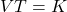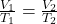Where;

• V1 and V2 represents the initial and final volumes respectively.
• T1 and T2 represents the initial and final temperatures respectively.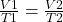Making V2 as the subject formula, we have;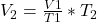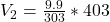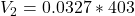Final volume, V2 = 13.18 Liters